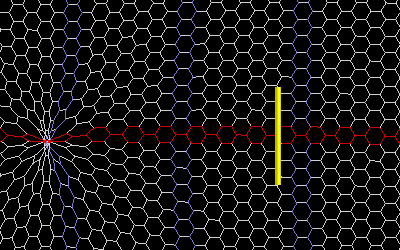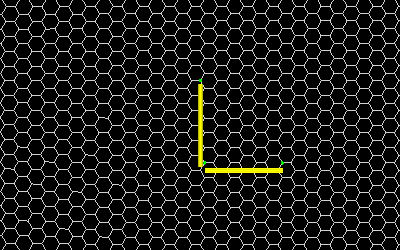top of page

"There are two kinds of truths: small truth and great truth. You can recognize a small truth because its opposite is a falsehood. The opposite of a great truth is another great truth."

- Niels Bohr -

## Gravitational Wave Detector

I have created some additional simulations to further demonstrate how gravitational waves travel through foamy ether. Figure 39 simulation shows a black hole (colored red) moving back and forth through the ether. The yellow cylinder represents a bar detector, or one of the arms of an 'interferometer based' gravitational wave detector (such as LIGO). The black hole moving through the ether causes a distortion (or wave) to propagate away from itself. If you carefully watch the ether movement on the top or bottom of the bar, you can see that the ether does indeed stretch along the length of the bar (as predicted by current gravitational wave theorists). This stretching, which is perpendicular to the direction of propagation, will cause the yellow bar to lengthen. Unfortunately though, the wavelength and speed of light will increase proportionately, so that the interferometer will not measure any changes in the length of its arms (as I explained earlier).Figure 39

Figure 40 simulation shows a gravitational wave passing by a Time Variance detector. In the simulation you can see that this wave also causes the ether to flow through the detector. This ether flow will cause time dilation to occur at each laser. But since the three lasers are far apart, they will experience time dilation at different phases. The simulation shows that the gravitational wave first hits the laser on the left; followed by the top laser, then finally the laser on the right. A spectrum analyzer, connected to these lasers via fiber optics, will sense the frequency fluctuations of the laser light. I created a cyan colored tracer on one of the nodes so that you can follow the flow of the ether more easily.Figure 40

Figure 41a simulation is similar to Figure 40 except that the black hole is made to move in a more elliptical orbit. The simulation shows that the gravitational wave in the foamy ether also follows an elliptical motion (as highlighted by the cyan tracer). For simplicity sake, I included only one black hole in the simulation. However in reality, compact objects (such as black holes and neutron stars) come in pairs and orbit each other.Figure 41a

Figure 41b is a plot of the changes in the horizontal position of the cyan tracer. This horizontal distortion in the ether is the gravitational wave.Figure 41b

Figure 41c is a plot of the changes in the vertical position of the cyan tracer. This vertical displacement is what's called an electromagnetic wave since the distortion is perpendicular to the direction of travel. The cells coloured in red show the EM wave more clearly.Figure 41c

Since the black holes take on an elliptical orbit, they emit both gravitational waves and electromagnetic waves (even thought they are electrically neutral). The 'to and from' motion create gravitational (compression) waves in the ether and the sideways motion create (transverse) electromagnetic waves.

Figure 42 shows a simulation of a gravitational wave passing through a typical interferometer type detector (such as LIGO). I made three of the nodes visible and colored them green. Then I created two yellow bars and arranged them in an L shaped configuration. The length of the bars were then programed to follow the distance between the green dots so that you can see how the bars stretch with the foamy ether. Unlike the previous three simulations, I forced the detector to 'follow along' with the ether so you can see how the length of the two bars change as the gravitational wave passes through.

But as I stated earlier, an interferometer will be unable to detect changes in the length of these arms because the speed of light increases as the ether stretches. This will result in the two returning beams of light to always be in phase!Figure 42

bottom of page